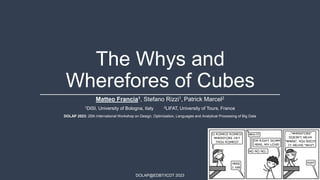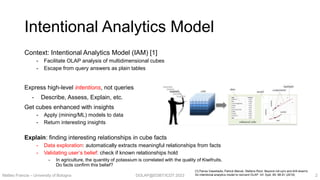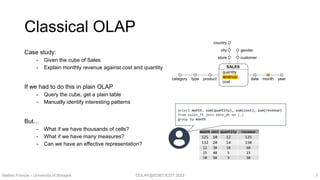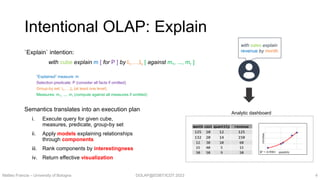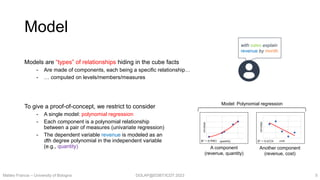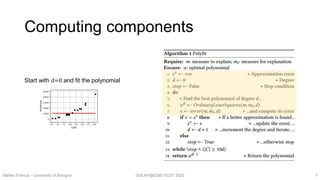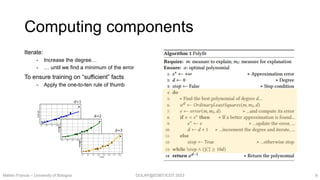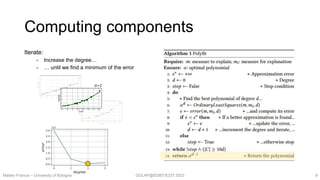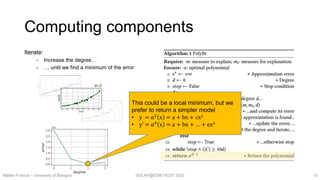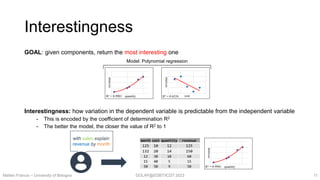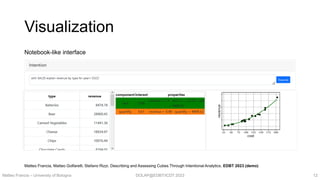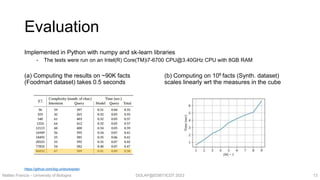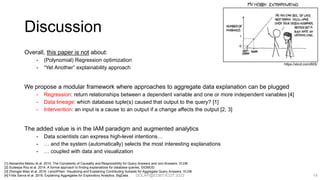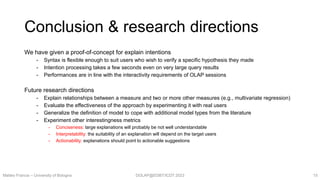1 of 16

### [DOLAP2023] The Whys and Wherefores of Cubes

1. DOLAP@EDBT/ICDT 2023 The Whys and Wherefores of Cubes Matteo Francia1, Stefano Rizzi1, Patrick Marcel2 1DISI, University of Bologna, Italy 2LIFAT, University of Tours, France DOLAP 2023: 25th International Workshop on Design, Optimization, Languages and Analytical Processing of Big Data
2. DOLAP@EDBT/ICDT 2023 Intentional Analytics Model Context: Intentional Analytics Model (IAM)  - Facilitate OLAP analysis of multidimensional cubes - Escape from query answers as plain tables Express high-level intentions, not queries - Describe, Assess, Explain, etc. Get cubes enhanced with insights - Apply (mining/ML) models to data - Return interesting insights Explain: finding interesting relationships in cube facts - Data exploration: automatically extracts meaningful relationships from facts - Validating user’s belief: check if known relationships hold - In agriculture, the quantity of potassium is correlated with the quality of Kiwifruits. Do facts confirm this belief? Matteo Francia – University of Bologna 2  Panos Vassiliadis, Patrick Marcel, Stefano Rizzi: Beyond roll-up's and drill-down's: An intentional analytics model to reinvent OLAP. Inf. Syst. 85: 68-91 (2019)
3. DOLAP@EDBT/ICDT 2023 Classical OLAP Case study: - Given the cube of Sales - Explain monthly revenue against cost and quantity If we had to do this in plain OLAP - Query the cube, get a plain table - Manually identify interesting patterns But… - What if we have thousands of cells? - What if we have many measures? - Can we have an effective representation? Matteo Francia – University of Bologna 3 select month, sum(quantity), sum(cost), sum(revenue) from sales_ft join date_dt on (…) group by month product type category customer gender store city country date month year quantity revenue cost SALES month cost quantity revenue 125 10 12 125 132 20 14 150 12 30 10 60 15 40 5 15 50 50 9 50
4. DOLAP@EDBT/ICDT 2023 Intentional OLAP: Explain `Explain` intention: with cube explain m [ for P ] by l1,…,ln [ against m1, ..., mr ] “Explained” measure: m Selection predicate: P (consider all facts if omitted) Group-by set: l1,…,ln (at least one level) Measures: m1, ..., mr (compute against all measures if omitted) Semantics translates into an execution plan i. Execute query for given cube, measures, predicate, group-by set ii. Apply models explaining relationships through components iii. Rank components by interestingness iv. Return effective visualization Matteo Francia – University of Bologna 4 with sales explain revenue by month Analytic dashboard R² = 0.9901 revenue quantity month cost quantity revenue 125 10 12 125 132 20 14 150 12 30 10 60 15 40 5 15 50 50 9 50
5. DOLAP@EDBT/ICDT 2023 Model Models are “types” of relationships hiding in the cube facts - Are made of components, each being a specific relationship… - … computed on levels/members/measures To give a proof-of-concept, we restrict to consider - A single model: polynomial regression - Each component is a polynomial relationship between a pair of measures (univariate regression) - The dependent variable revenue is modeled as an dth degree polynomial in the independent variable (e.g., quantity) Matteo Francia – University of Bologna 5 R² = 0.9901 revenue quantity R² = 0.6524 revenue cost Model: Polynomial regression A component (revenue, quantity) Another component (revenue, cost) with sales explain revenue by month
6. DOLAP@EDBT/ICDT 2023 Components Each component is a polynomial relationship αd ( ) between a pair of measures - How to choose the “best” polynomial and avoid overfitting? - E.g., consider revenue = αd (𝑐𝑜𝑠𝑡) We need an error function weighting the degree (d): fact αd fact.m −fact.m 2 facts −d −1 - αd ( ) is the polynomial with degree d fitted with OrdinaryLeastSquares method - The error is computed against a test set containing 30% of the facts Matteo Francia – University of Bologna 6 Too simple (high error, low polynomial degree) Too complex (lower error, higher degree)
7. DOLAP@EDBT/ICDT 2023 Computing components Matteo Francia – University of Bologna 7 Start with d=0 and fit the polynomial
8. DOLAP@EDBT/ICDT 2023 Iterate: - Increase the degree… - … until we find a minimum of the error To ensure training on “sufficient” facts - Apply the one-to-ten rule of thumb d=1 d=2 d=3 Computing components Matteo Francia – University of Bologna 8
9. DOLAP@EDBT/ICDT 2023 Computing components Matteo Francia – University of Bologna 9 Iterate: - Increase the degree… - … until we find a minimum of the error d=2
10. DOLAP@EDBT/ICDT 2023 Computing components Matteo Francia – University of Bologna 10 Iterate: - Increase the degree… - … until we find a minimum of the error d=2 This could be a local minimum, but we prefer to return a simpler model • y = α2 x = a + bx + cx2 • y’ = α4 x = a + bx + … + ex4
11. DOLAP@EDBT/ICDT 2023 Interestingness GOAL: given components, return the most interesting one Interestingness: how variation in the dependent variable is predictable from the independent variable - This is encoded by the coefficient of determination R2 - The better the model, the closer the value of R2 to 1 Matteo Francia – University of Bologna 11 R² = 0.9901 revenue quantity R² = 0.6524 revenue cost Model: Polynomial regression with sales explain revenue by month R² = 0.9901 revenue quantity month cost quantity revenue 125 10 12 125 132 20 14 150 12 30 10 60 15 40 5 15 50 50 9 50
12. DOLAP@EDBT/ICDT 2023 Visualization Matteo Francia – University of Bologna 12 Matteo Francia, Matteo Golfarelli, Stefano Rizzi. Describing and Assessing Cubes Through Intentional Analytics. EDBT 2023 (demo) Notebook-like interface
13. DOLAP@EDBT/ICDT 2023 (b) Computing on 106 facts (Synth. dataset) scales linearly wrt the measures in the cube Evaluation (a) Computing the results on ~90K facts (Foodmart dataset) takes 0.5 seconds Matteo Francia – University of Bologna 13 Implemented in Python with numpy and sk-learn libraries - The tests were run on an Intel(R) Core(TM)i7-6700 CPU@3.40GHz CPU with 8GB RAM https://github.com/big-unibo/explain
14. DOLAP@EDBT/ICDT 2023 Discussion Overall, this paper is not about: - (Polynomial) Regression optimization - “Yet Another” explainability approach We propose a modular framework where approaches to aggregate data explanation can be plugged - Regression: return relationships between a dependent variable and one or more independent variables  - Data lineage: which database tuple(s) caused that output to the query?  - Intervention: an input is a cause to an output if a change affects the output [2, 3] The added value is in the IAM paradigm and augmented analytics - Data scientists can express high-level intentions… - … and the system (automatically) selects the most interesting explanations - … coupled with data and visualization 14  Alexandra Meliou et al. 2010. The Complexity of Causality and Responsibility for Query Answers and non-Answers. VLDB  Sudeepa Roy et al. 2014. A formal approach to finding explanations for database queries. SIGMOD  Zhengjie Miao et al. 2019. LensXPlain: Visualizing and Explaining Contributing Subsets for Aggregate Query Answers. VLDB  Fotis Savva et al. 2018. Explaining Aggregates for Exploratory Analytics. BigData. https://xkcd.com/605/
15. DOLAP@EDBT/ICDT 2023 Conclusion & research directions We have given a proof-of-concept for explain intentions - Syntax is flexible enough to suit users who wish to verify a specific hypothesis they made - Intention processing takes a few seconds even on very large query results - Performances are in line with the interactivity requirements of OLAP sessions Future research directions - Explain relationships between a measure and two or more other measures (e.g., multivariate regression) - Evaluate the effectiveness of the approach by experimenting it with real users - Generalize the definition of model to cope with additional model types from the literature - Experiment other interestingness metrics - Conciseness: large explanations will probably be not well understandable - Interpretability: the suitability of an explanation will depend on the target users - Actionability: explanations should point to actionable suggestions Matteo Francia – University of Bologna 15
16. DOLAP@EDBT/ICDT 2023 Questions? Matteo Francia – University of Bologna 16 Thank you.

### Editor's Notes

1. The Intentional Analytics Model (IAM) has been envisioned as a way to tightly couple OLAP and analytics by (i) letting users explore multidimensional cubes stating their intentions, and (ii) returning multidimensional data coupled with knowledge in- sights in the form of annotations of subsets of data
2. average squared difference between the observed and predicted values. When a model has no error, the MSE equals zero.